Elementary Algebra

# 2.4Use a General Strategy to Solve Linear Equations

Elementary Algebra2.4 Use a General Strategy to Solve Linear Equations

### Learning Objectives

By the end of this section, you will be able to:

• Solve equations using a general strategy
• Classify equations

### Be Prepared 2.4

Before you get started, take this readiness quiz.

1. Simplify: $−(a−4).−(a−4).$
If you missed this problem, review Example 1.137.
2. Multiply: $32(12x+20)32(12x+20)$.
If you missed this problem, review Example 1.133.
3. Simplify: $5−2(n+1)5−2(n+1)$.
If you missed this problem, review Example 1.138.
4. Multiply: $3(7y+9)3(7y+9)$.
If you missed this problem, review Example 1.132.
5. Multiply: $(2.5)(6.4)(2.5)(6.4)$.
If you missed this problem, review Example 1.97.

### Solve Equations Using the General Strategy

Until now we have dealt with solving one specific form of a linear equation. It is time now to lay out one overall strategy that can be used to solve any linear equation. Some equations we solve will not require all these steps to solve, but many will.

Beginning by simplifying each side of the equation makes the remaining steps easier.

### Example 2.37

#### How to Solve Linear Equations Using the General Strategy

Solve: $−6(x+3)=24.−6(x+3)=24.$

### Try It 2.73

Solve: $5(x+3)=35.5(x+3)=35.$

### Try It 2.74

Solve: $6(y−4)=−18.6(y−4)=−18.$

### How To

#### General strategy for solving linear equations.

1. Step 1. Simplify each side of the equation as much as possible.
Use the Distributive Property to remove any parentheses.
Combine like terms.
2. Step 2. Collect all the variable terms on one side of the equation.
Use the Addition or Subtraction Property of Equality.
3. Step 3. Collect all the constant terms on the other side of the equation.
Use the Addition or Subtraction Property of Equality.
4. Step 4. Make the coefficient of the variable term to equal to 1.
Use the Multiplication or Division Property of Equality.
State the solution to the equation.
5. Step 5. Check the solution. Substitute the solution into the original equation to make sure the result is a true statement.

### Example 2.38

Solve: $−(y+9)=8.−(y+9)=8.$

### Try It 2.75

Solve: $−(y+8)=−2.−(y+8)=−2.$

### Try It 2.76

Solve: $−(z+4)=−12.−(z+4)=−12.$

### Example 2.39

Solve: $5(a−3)+5=−105(a−3)+5=−10$.

### Try It 2.77

Solve: $2(m−4)+3=−12(m−4)+3=−1$.

### Try It 2.78

Solve: $7(n−3)−8=−157(n−3)−8=−15$.

### Example 2.40

Solve: $23(6m−3)=8−m23(6m−3)=8−m$.

### Try It 2.79

Solve: $13(6u+3)=7−u13(6u+3)=7−u$.

### Try It 2.80

Solve: $23(9x−12)=8+2x23(9x−12)=8+2x$.

### Example 2.41

Solve: $8−2(3y+5)=08−2(3y+5)=0$.

### Try It 2.81

Solve: $12−3(4j+3)=−1712−3(4j+3)=−17$.

### Try It 2.82

Solve: $−6−8(k−2)=−10−6−8(k−2)=−10$.

### Example 2.42

Solve: $4(x−1)−2=5(2x+3)+64(x−1)−2=5(2x+3)+6$.

### Try It 2.83

Solve: $6(p−3)−7=5(4p+3)−126(p−3)−7=5(4p+3)−12$.

### Try It 2.84

Solve: $8(q+1)−5=3(2q−4)−18(q+1)−5=3(2q−4)−1$.

### Example 2.43

Solve: $10[3−8(2s−5)]=15(40−5s)10[3−8(2s−5)]=15(40−5s)$.

### Try It 2.85

Solve: $6[4−2(7y−1)]=8(13−8y)6[4−2(7y−1)]=8(13−8y)$.

### Try It 2.86

Solve: $12[1−5(4z−1)]=3(24+11z)12[1−5(4z−1)]=3(24+11z)$.

### Example 2.44

Solve: $0.36(100n+5)=0.6(30n+15)0.36(100n+5)=0.6(30n+15)$.

### Try It 2.87

Solve: $0.55(100n+8)=0.6(85n+14)0.55(100n+8)=0.6(85n+14)$.

### Try It 2.88

Solve: $0.15(40m−120)=0.5(60m+12)0.15(40m−120)=0.5(60m+12)$.

### Classify Equations

Consider the equation we solved at the start of the last section, $7x+8=−137x+8=−13$. The solution we found was $x=−3x=−3$. This means the equation $7x+8=−137x+8=−13$ is true when we replace the variable, x, with the value $−3−3$. We showed this when we checked the solution $x=−3x=−3$ and evaluated $7x+8=−137x+8=−13$ for $x=−3x=−3$.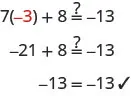If we evaluate $7x+87x+8$ for a different value of x, the left side will not be $−13−13$.

The equation $7x+8=−137x+8=−13$ is true when we replace the variable, x, with the value $−3−3$, but not true when we replace x with any other value. Whether or not the equation $7x+8=−137x+8=−13$ is true depends on the value of the variable. Equations like this are called conditional equations.

All the equations we have solved so far are conditional equations.

### Conditional equation

An equation that is true for one or more values of the variable and false for all other values of the variable is a conditional equation.

Now let’s consider the equation $2y+6=2(y+3)2y+6=2(y+3)$. Do you recognize that the left side and the right side are equivalent? Let’s see what happens when we solve for y.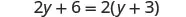Distribute.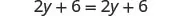Subtract $2y2y$ to get the $yy$’s to one side.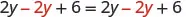Simplify—the $yy$’s are gone!But $6=66=6$ is true.

This means that the equation $2y+6=2(y+3)2y+6=2(y+3)$ is true for any value of y. We say the solution to the equation is all of the real numbers. An equation that is true for any value of the variable like this is called an identity.

### Identity

An equation that is true for any value of the variable is called an identity.

The solution of an identity is all real numbers.

What happens when we solve the equation $5z=5z−15z=5z−1$?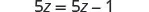Subtract $5z5z$ to get the constant alone on the right.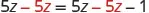Simplify—the $zz$’s are gone!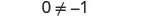But $0≠−10≠−1$.

Solving the equation $5z=5z−15z=5z−1$ led to the false statement $0=−10=−1$. The equation $5z=5z−15z=5z−1$ will not be true for any value of z. It has no solution. An equation that has no solution, or that is false for all values of the variable, is called a contradiction.

An equation that is false for all values of the variable is called a contradiction.

### Example 2.45

Classify the equation as a conditional equation, an identity, or a contradiction. Then state the solution.

$6(2n−1)+3=2n−8+5(2n+1)6(2n−1)+3=2n−8+5(2n+1)$

### Try It 2.89

Classify the equation as a conditional equation, an identity, or a contradiction and then state the solution:

$4+9(3x−7)=−42x−13+23(3x−2)4+9(3x−7)=−42x−13+23(3x−2)$

### Try It 2.90

Classify the equation as a conditional equation, an identity, or a contradiction and then state the solution:

$8(1−3x)+15(2x+7)=2(x+50)+4(x+3)+18(1−3x)+15(2x+7)=2(x+50)+4(x+3)+1$

### Example 2.46

Classify as a conditional equation, an identity, or a contradiction. Then state the solution.

$10+4(p−5)=010+4(p−5)=0$

### Try It 2.91

Classify the equation as a conditional equation, an identity, or a contradiction and then state the solution: $11(q+3)−5=1911(q+3)−5=19$

### Try It 2.92

Classify the equation as a conditional equation, an identity, or a contradiction and then state the solution: $6+14(k−8)=956+14(k−8)=95$

### Example 2.47

Classify the equation as a conditional equation, an identity, or a contradiction. Then state the solution.

$5m+3(9+3m)=2(7m−11)5m+3(9+3m)=2(7m−11)$

### Try It 2.93

Classify the equation as a conditional equation, an identity, or a contradiction and then state the solution:

$12c+5(5+3c)=3(9c−4)12c+5(5+3c)=3(9c−4)$

### Try It 2.94

Classify the equation as a conditional equation, an identity, or a contradiction and then state the solution:

$4(7d+18)=13(3d−2)−11d4(7d+18)=13(3d−2)−11d$

Type of equation What happens when you solve it? Solution
Conditional Equation True for one or more values of the variables and false for all other values One or more values
Identity True for any value of the variable All real numbers
Contradiction False for all values of the variable No solution
Table 2.5

### Section 2.4 Exercises

#### Practice Makes Perfect

Solve Equations Using the General Strategy for Solving Linear Equations

In the following exercises, solve each linear equation.

232.

$15 ( y − 9 ) = −60 15 ( y − 9 ) = −60$

233.

$21 ( y − 5 ) = −42 21 ( y − 5 ) = −42$

234.

$−9 ( 2 n + 1 ) = 36 −9 ( 2 n + 1 ) = 36$

235.

$−16 ( 3 n + 4 ) = 32 −16 ( 3 n + 4 ) = 32$

236.

$8 ( 22 + 11 r ) = 0 8 ( 22 + 11 r ) = 0$

237.

$5 ( 8 + 6 p ) = 0 5 ( 8 + 6 p ) = 0$

238.

$− ( w − 12 ) = 30 − ( w − 12 ) = 30$

239.

$− ( t − 19 ) = 28 − ( t − 19 ) = 28$

240.

$9 ( 6 a + 8 ) + 9 = 81 9 ( 6 a + 8 ) + 9 = 81$

241.

$8 ( 9 b − 4 ) − 12 = 100 8 ( 9 b − 4 ) − 12 = 100$

242.

$32 + 3 ( z + 4 ) = 41 32 + 3 ( z + 4 ) = 41$

243.

$21 + 2 ( m − 4 ) = 25 21 + 2 ( m − 4 ) = 25$

244.

$51 + 5 ( 4 − q ) = 56 51 + 5 ( 4 − q ) = 56$

245.

$−6 + 6 ( 5 − k ) = 15 −6 + 6 ( 5 − k ) = 15$

246.

$2 ( 9 s − 6 ) − 62 = 16 2 ( 9 s − 6 ) − 62 = 16$

247.

$8 ( 6 t − 5 ) − 35 = −27 8 ( 6 t − 5 ) − 35 = −27$

248.

$3 ( 10 − 2 x ) + 54 = 0 3 ( 10 − 2 x ) + 54 = 0$

249.

$−2 ( 11 − 7 x ) + 54 = 4 −2 ( 11 − 7 x ) + 54 = 4$

250.

$2 3 ( 9 c − 3 ) = 22 2 3 ( 9 c − 3 ) = 22$

251.

$3 5 ( 10 x − 5 ) = 27 3 5 ( 10 x − 5 ) = 27$

252.

$1 5 ( 15 c + 10 ) = c + 7 1 5 ( 15 c + 10 ) = c + 7$

253.

$1 4 ( 20 d + 12 ) = d + 7 1 4 ( 20 d + 12 ) = d + 7$

254.

$18 − ( 9 r + 7 ) = −16 18 − ( 9 r + 7 ) = −16$

255.

$15 − ( 3 r + 8 ) = 28 15 − ( 3 r + 8 ) = 28$

256.

$5 − ( n − 1 ) = 19 5 − ( n − 1 ) = 19$

257.

$−3 − ( m − 1 ) = 13 −3 − ( m − 1 ) = 13$

258.

$11 − 4 ( y − 8 ) = 43 11 − 4 ( y − 8 ) = 43$

259.

$18 − 2 ( y − 3 ) = 32 18 − 2 ( y − 3 ) = 32$

260.

$24 − 8 ( 3 v + 6 ) = 0 24 − 8 ( 3 v + 6 ) = 0$

261.

$35 − 5 ( 2 w + 8 ) = −10 35 − 5 ( 2 w + 8 ) = −10$

262.

$4 ( a − 12 ) = 3 ( a + 5 ) 4 ( a − 12 ) = 3 ( a + 5 )$

263.

$−2 ( a − 6 ) = 4 ( a − 3 ) −2 ( a − 6 ) = 4 ( a − 3 )$

264.

$2 ( 5 − u ) = −3 ( 2 u + 6 ) 2 ( 5 − u ) = −3 ( 2 u + 6 )$

265.

$5 ( 8 − r ) = −2 ( 2 r − 16 ) 5 ( 8 − r ) = −2 ( 2 r − 16 )$

266.

$3 ( 4 n − 1 ) − 2 = 8 n + 3 3 ( 4 n − 1 ) − 2 = 8 n + 3$

267.

$9 ( 2 m − 3 ) − 8 = 4 m + 7 9 ( 2 m − 3 ) − 8 = 4 m + 7$

268.

$12 + 2 ( 5 − 3 y ) = −9 ( y − 1 ) − 2 12 + 2 ( 5 − 3 y ) = −9 ( y − 1 ) − 2$

269.

$−15 + 4 ( 2 − 5 y ) = −7 ( y − 4 ) + 4 −15 + 4 ( 2 − 5 y ) = −7 ( y − 4 ) + 4$

270.

$8 ( x − 4 ) − 7 x = 14 8 ( x − 4 ) − 7 x = 14$

271.

$5 ( x − 4 ) − 4 x = 14 5 ( x − 4 ) − 4 x = 14$

272.

$5 + 6 ( 3 s − 5 ) = −3 + 2 ( 8 s − 1 ) 5 + 6 ( 3 s − 5 ) = −3 + 2 ( 8 s − 1 )$

273.

$−12 + 8 ( x − 5 ) = −4 + 3 ( 5 x − 2 ) −12 + 8 ( x − 5 ) = −4 + 3 ( 5 x − 2 )$

274.

$4 ( u − 1 ) − 8 = 6 ( 3 u − 2 ) − 7 4 ( u − 1 ) − 8 = 6 ( 3 u − 2 ) − 7$

275.

$7 ( 2 n − 5 ) = 8 ( 4 n − 1 ) − 9 7 ( 2 n − 5 ) = 8 ( 4 n − 1 ) − 9$

276.

$4 ( p − 4 ) − ( p + 7 ) = 5 ( p − 3 ) 4 ( p − 4 ) − ( p + 7 ) = 5 ( p − 3 )$

277.

$3 ( a − 2 ) − ( a + 6 ) = 4 ( a − 1 ) 3 ( a − 2 ) − ( a + 6 ) = 4 ( a − 1 )$

278.

$− ( 9 y + 5 ) − ( 3 y − 7 ) − ( 9 y + 5 ) − ( 3 y − 7 )$
$= 16 − ( 4 y − 2 ) = 16 − ( 4 y − 2 )$

279.

$− ( 7 m + 4 ) − ( 2 m − 5 ) − ( 7 m + 4 ) − ( 2 m − 5 )$
$= 14 − ( 5 m − 3 ) = 14 − ( 5 m − 3 )$

280.

$4 [ 5 − 8 ( 4 c − 3 ) ] 4 [ 5 − 8 ( 4 c − 3 ) ]$
$= 12 ( 1 − 13 c ) − 8 = 12 ( 1 − 13 c ) − 8$

281.

$5 [ 9 − 2 ( 6 d − 1 ) ] 5 [ 9 − 2 ( 6 d − 1 ) ]$
$= 11 ( 4 − 10 d ) − 139 = 11 ( 4 − 10 d ) − 139$

282.

$3 [ −9 + 8 ( 4 h − 3 ) ] 3 [ −9 + 8 ( 4 h − 3 ) ]$
$= 2 ( 5 − 12 h ) − 19 = 2 ( 5 − 12 h ) − 19$

283.

$3 [ −14 + 2 ( 15 k − 6 ) ] 3 [ −14 + 2 ( 15 k − 6 ) ]$
$= 8 ( 3 − 5 k ) − 24 = 8 ( 3 − 5 k ) − 24$

284.

$5 [ 2 ( m + 4 ) + 8 ( m − 7 ) ] 5 [ 2 ( m + 4 ) + 8 ( m − 7 ) ]$
$= 2 [ 3 ( 5 + m ) − ( 21 − 3 m ) ] = 2 [ 3 ( 5 + m ) − ( 21 − 3 m ) ]$

285.

$10 [ 5 ( n + 1 ) + 4 ( n − 1 ) ] 10 [ 5 ( n + 1 ) + 4 ( n − 1 ) ]$
$= 11 [ 7 ( 5 + n ) − ( 25 − 3 n ) ] = 11 [ 7 ( 5 + n ) − ( 25 − 3 n ) ]$

286.

$5 ( 1.2 u − 4.8 ) = −12 5 ( 1.2 u − 4.8 ) = −12$

287.

$4 ( 2.5 v − 0.6 ) = 7.6 4 ( 2.5 v − 0.6 ) = 7.6$

288.

$0.25 ( q − 6 ) = 0.1 ( q + 18 ) 0.25 ( q − 6 ) = 0.1 ( q + 18 )$

289.

$0.2 ( p − 6 ) = 0.4 ( p + 14 ) 0.2 ( p − 6 ) = 0.4 ( p + 14 )$

290.

$0.2 ( 30 n + 50 ) = 28 0.2 ( 30 n + 50 ) = 28$

291.

$0.5 ( 16 m + 34 ) = −15 0.5 ( 16 m + 34 ) = −15$

Classify Equations

In the following exercises, classify each equation as a conditional equation, an identity, or a contradiction and then state the solution.

292.

$23 z + 19 = 3 ( 5 z − 9 ) + 8 z + 46 23 z + 19 = 3 ( 5 z − 9 ) + 8 z + 46$

293.

$15 y + 32 = 2 ( 10 y − 7 ) − 5 y + 46 15 y + 32 = 2 ( 10 y − 7 ) − 5 y + 46$

294.

$5 ( b − 9 ) + 4 ( 3 b + 9 ) = 6 ( 4 b − 5 ) − 7 b + 21 5 ( b − 9 ) + 4 ( 3 b + 9 ) = 6 ( 4 b − 5 ) − 7 b + 21$

295.

$9 ( a − 4 ) + 3 ( 2 a + 5 ) = 7 ( 3 a − 4 ) − 6 a + 7 9 ( a − 4 ) + 3 ( 2 a + 5 ) = 7 ( 3 a − 4 ) − 6 a + 7$

296.

$18 ( 5 j − 1 ) + 29 = 47 18 ( 5 j − 1 ) + 29 = 47$

297.

$24 ( 3 d − 4 ) + 100 = 52 24 ( 3 d − 4 ) + 100 = 52$

298.

$22 ( 3 m − 4 ) = 8 ( 2 m + 9 ) 22 ( 3 m − 4 ) = 8 ( 2 m + 9 )$

299.

$30 ( 2 n − 1 ) = 5 ( 10 n + 8 ) 30 ( 2 n − 1 ) = 5 ( 10 n + 8 )$

300.

$7 v + 42 = 11 ( 3 v + 8 ) − 2 ( 13 v − 1 ) 7 v + 42 = 11 ( 3 v + 8 ) − 2 ( 13 v − 1 )$

301.

$18 u − 51 = 9 ( 4 u + 5 ) − 6 ( 3 u − 10 ) 18 u − 51 = 9 ( 4 u + 5 ) − 6 ( 3 u − 10 )$

302.

$3 ( 6 q − 9 ) + 7 ( q + 4 ) = 5 ( 6 q + 8 ) − 5 ( q + 1 ) 3 ( 6 q − 9 ) + 7 ( q + 4 ) = 5 ( 6 q + 8 ) − 5 ( q + 1 )$

303.

$5 ( p + 4 ) + 8 ( 2 p − 1 ) = 9 ( 3 p − 5 ) − 6 ( p − 2 ) 5 ( p + 4 ) + 8 ( 2 p − 1 ) = 9 ( 3 p − 5 ) − 6 ( p − 2 )$

304.

$12 ( 6 h − 1 ) = 8 ( 8 h + 5 ) − 4 12 ( 6 h − 1 ) = 8 ( 8 h + 5 ) − 4$

305.

$9 ( 4 k − 7 ) = 11 ( 3 k + 1 ) + 4 9 ( 4 k − 7 ) = 11 ( 3 k + 1 ) + 4$

306.

$45 ( 3 y − 2 ) = 9 ( 15 y − 6 ) 45 ( 3 y − 2 ) = 9 ( 15 y − 6 )$

307.

$60 ( 2 x − 1 ) = 15 ( 8 x + 5 ) 60 ( 2 x − 1 ) = 15 ( 8 x + 5 )$

308.

$16 ( 6 n + 15 ) = 48 ( 2 n + 5 ) 16 ( 6 n + 15 ) = 48 ( 2 n + 5 )$

309.

$36 ( 4 m + 5 ) = 12 ( 12 m + 15 ) 36 ( 4 m + 5 ) = 12 ( 12 m + 15 )$

310.

$9 ( 14 d + 9 ) + 4 d = 13 ( 10 d + 6 ) + 3 9 ( 14 d + 9 ) + 4 d = 13 ( 10 d + 6 ) + 3$

311.

$11 ( 8 c + 5 ) − 8 c = 2 ( 40 c + 25 ) + 5 11 ( 8 c + 5 ) − 8 c = 2 ( 40 c + 25 ) + 5$

#### Everyday Math

312.

Fencing Micah has 44 feet of fencing to make a dog run in his yard. He wants the length to be 2.5 feet more than the width. Find the length, L, by solving the equation $2L+2(L−2.5)=442L+2(L−2.5)=44$.

313.

Coins Rhonda has \$1.90 in nickels and dimes. The number of dimes is one less than twice the number of nickels. Find the number of nickels, n, by solving the equation $0.05n+0.10(2n−1)=1.900.05n+0.10(2n−1)=1.90$.

#### Writing Exercises

314.

Using your own words, list the steps in the general strategy for solving linear equations.

315.

Explain why you should simplify both sides of an equation as much as possible before collecting the variable terms to one side and the constant terms to the other side.

316.

What is the first step you take when solving the equation $3−7(y−4)=383−7(y−4)=38$ ? Why is this your first step?

317.

Solve the equation $14(8x+20)=3x−414(8x+20)=3x−4$ explaining all the steps of your solution as in the examples in this section.

#### Self Check

After completing the exercises, use this checklist to evaluate your mastery of the objective of this section.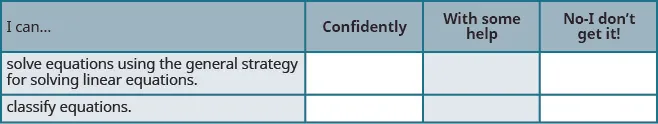On a scale of 1-10, how would you rate your mastery of this section in light of your responses on the checklist? How can you improve this?

Order a print copy

As an Amazon Associate we earn from qualifying purchases.